# Methods and formulas for Box-Cox

## Box-Cox formula

If you use a Box-Cox transformation, Minitab transforms the original data values (Yi) according to the following formula:

where λ is the parameter for the transformation. Minitab then creates a control chart of the transformed data values (Wi). To learn how Minitab chooses the optimal value for λ, go to Methods and formulas for Box-Cox Transformation.

## Common λ values

The following table shows some commonly used λ values and their transformations.
λ Transformation
2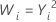0.5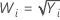0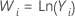−0.5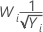−1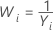By using this site you agree to the use of cookies for analytics and personalized content.  Read our policy